Date: Wed, 16 Sep 1998 17:59:28 -0600 (CST) Subject: Probability Question Name: Chris Who is asking: Student Level: Middle Question: Six marbles are placed in one of three boxes. What is the probability that each box contains two marbles ? What is the formula used ? Hi Chris, There are at least two different ways to interpret this question. The question might be interpreted to mean that we are to place 6 identical marbles in 3 boxes labeled A, B and C. Here, for each marble there are three choices for its placement, either in box A, B or C. Thus there are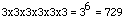ways to place the 6 marbles in the 3 boxes. If you know about permutations and combinations then you see that there are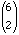ways to select 2 balls for box A, and for each selection there are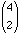ways to select 2 of the remaining balls for box B, and finally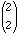ways to select the last 2 balls for box C. Thus there are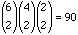ways to put two balls in each box.    If you don't know about permutations and combinations you can argue as follows. Suppose that you are going to select the balls one at a time and put the first you select in box A, the second in box A, the third in box B, the fourth in box B and so on. There are 6 way to select the first ball, 5 to select the second, 4 to select the third and so on, so there are 6x5x4x3x2x1=6! ways to select the balls one at a time. Once you have such an arrangement you can switch the order of the 2 balls in box A to obtain an indistinguishable arrangement. The same is true for boxes B and C so each distinguishable arrangement appears 8 time. Hence there are 6!/8=90 ways to put two balls in each box.    Thus for this interpretation of the question the probability is 90/729=10/81. If we suppose the problem means 6 identical marbles into 3 identical boxes we can answer in the following manner. We will use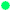to represent a marble. If we line up 6 marbles the define 7 spaces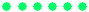5 between the marbles and 1 at each end. Thus if we wanted to divide the marbles into only two boxes all we would have to do is place a divider in one of the 7 spots, 7 ways to do this. For example if we use a red bar as the divider we might see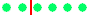or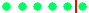or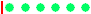these would represent a division of 2 marbles in the first box and 4 in the second, 5 marbles in the first box and 1 in the second, and 0 marbles in the first box and 6 in the second.    Our problem is more complicated in that we have three boxes which means we need two dividers, a red bar and a blue bar. In this notation an arrangement such as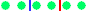for example means 2 marbles in the first box, 2 in the second and 2 in the third while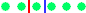means 2 in the first, 1 in the second and 3 in the third, and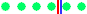would mean 4 in the first, none in the second and 2 in the third.    Our problem is then, how many ways can we put in the two dividers? Again if you know about permutations and combinations there is an easy way to see the answer is 28 but let's just do it step by step. First place one of the dividers, say the red bar as we did above. Where can we place the second? Let's have another look at the situation after the red bar has beeen placed -- we now have 7 objects lined up, the 6 marbles and the divider. These determine 8 spaces, 1 between any pair of adjacent objects and 1 at each end. For exampleNotice that there is a space on either side of the red bar. So where can we place the blue bar? In any of the 8 places! For examplegiving us a division of 2, 1 and 3 marbles. It looks like there are 7 x 8 = 56 possiblities but we must be careful! Look at the pairs of solutions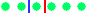they each give 2 marbles in the first box, 1 in the second and 3 in the third, so we've counted this answer twice -- this is because just switching the red and the blue bars doesn't give us a new answer. This means all told there are (7*8)/2 = 28 different possiblities of which the situation with 2 marbles in the first box, 2 in the second and 2 in the third only appears once. Therefore, with this interpretation of the problem the probability is 1/28 that each box gets exactly 2 marbles. Cheers, Penny and Chris
Go to Math Central

To return to the previous page use your browser's back button.